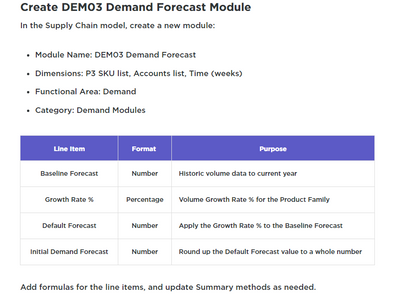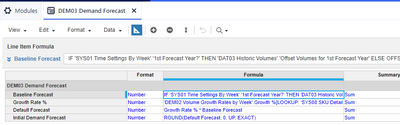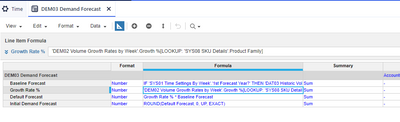# DEM 03 Baseline forecast formulasHi,

I am trying to write formulas for this activity:Here is what I am doing:But the results are nor correct,

How should I change the formulas and/or summary methods?

Thanks

Tagged:

•1. Default Forecast Line item has to be Baseline Forecast * (1+Growth Rate)

2. Growth Rate has to be Closing Balance on Time for Summary methods.

Hope that helps

Misbah

Miz Logix

•It works for default forcast. Thanks. But I dont understand why to +1 in this formulas: Baseline Forecast * (1+Growth Rate)

And it is not still working correctly for Initial demand forecast. The result for specific item should be 90 but mine is 88. It seems that ROUND doesnt work correctly.( default forecast is 87.46)

•I think as we have 2 forecast year and the wrong amount is for second one I need to make changes in baseline forecast formulas

•For Default Forecast it has to be uplifted, thats why 1 in the formula. For example if the baseline is 100 and you want to uplift it by 1% you can not multiply 100 with 1 that is going to be give you 1 as result. Expectation is to have 101 in the result which can be achieved only when you say Baseline Forecast + Baseline Forecast * Growth Rate or Baseline Forecast(1+ Growth Rate). That's Math!

For this one, please check your numbers in the Baseline Forecast Line and verify if those numbers are right

Misbah

Miz Logix